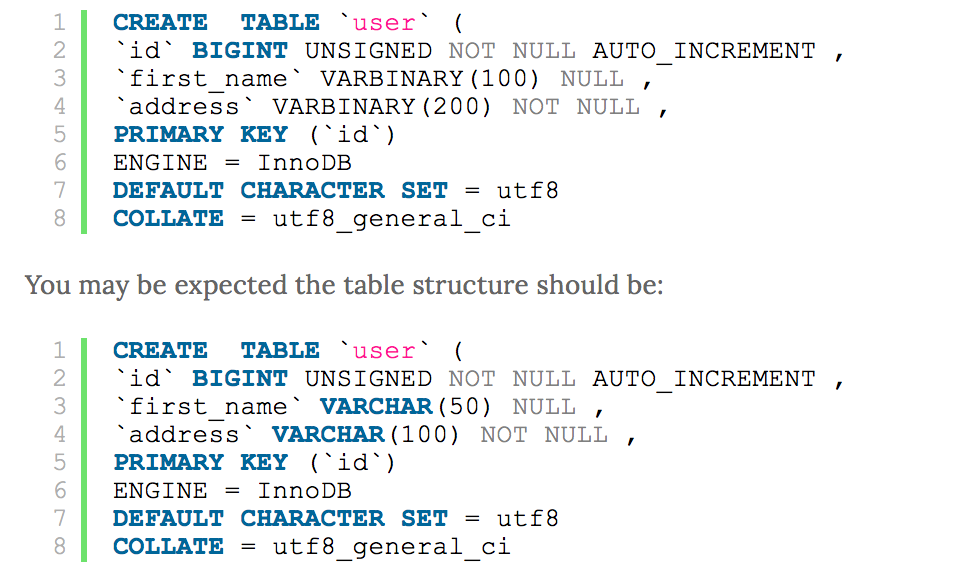top of page

# Encrypting MySQL's DB

AES Encryption

Sometimes clients want that the information they collected from the user should be encrypted and stored in database. Data encryption and decryption is a common technique for secured data. In this article I’ll show how could you use mysql’s built in function to encrypt and decrypt data.

Suppose you’ve a table where you want to encrypt user’s name & address. So look below the structure of the table. In this table we will store name and address as encrypted.To encrypt & decrypt mysql data we will use AES_ENCRYPT() and AES_DECRYPT() functions. These functions used the official AES (Advanced Encryption Standard) algorithm & encode data with a 128-bit key length. 128 bits is much faster and secure enough for most purposes.

Why we used VARBINARY data type instead of VARCHAR:

Because AES_ENCRYPT() encrypts a string and returns a binary string. AES_DECRYPT() decrypts the encrypted string and returns the original string.

AES is a block-level algorithm. So when data encrypted it is padded. So you can calculate the length of the result string using this formula:

1. 16 × (trunc(string_length / 16) + 1)

So if your address field structure is = VARCHAR(100) ; //100 length of varchar Then before your encryption it should be converted = 16 * (trunc(100/ 16) + 1) = 16 * (6.25 + 1) = 16 * 7.25 = 116

So VARCHAR(100) should be converted to VARBINARY(116) minimum. I suggest use little more like VARBINARY(150) in this case.

Because if AES_DECRYPT() detects invalid data or incorrect padding, it will return NULL. But it is also possible for AES_DECRYPT() to return a non-NULL value (possibly garbage) if the input data or the key is invalid.

Syntax:

1| AES_ENCRYPT(str, key_str);

2| AES_DECRYPT(crypt_str,key_str);

Please remember, the encryption and decryption will occur based on a key. So you’ve to keep that key in a secret place and using variable you could pass the key to mysql to encry

pt and decrypt data.

Now look how I insert data using AES_ENCRYPT, where I used key ‘usa2010’ :

INSERT into user (first_name, address) VALUES (AES_ENCRYPT('Obama', 'usa2010'),AES_ENCRYPT('Obama', 'usa2010'));

Now look how I decrypt data using AES_DECRYPT:

SELECT AES_DECRYPT(first_name, 'usa2010'), AES_DECRYPT(address, 'usa2010') from user;

AES_ENCRYPT() and AES_DECRYPT() can be considered the most cryptographically secure encryption functions currently available in MySQL.

No tags yet.
bottom of page